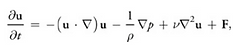# 2D fluid simulation

This is the final project I made for the Physics-based animation class during my school. The code is placed here.

I have implemented a 2D stable fluid method using OpenGl based on the NVIDIA article and this paper

I'll omit the detailed explanation since there is a detailed theory explanation on the NVIDIA article.

However, if I simply explained it, this program is to simulate Naiver-Stokes Equation in continuous small-time (I know this sounds dumb, but I don't really know how to explain this otherwise XD)Pressure

Diffusion

External ForcesThere are 4 terms in this equation, Advection, Pressure, Diffusion, and External Forces. For each iteration, I stored those states as textures, and update the final picture based on the textures every frame. Below the transition of textures in 1 frame (I skipped the diffusion part for my implementation since it looks more natural to me)Current frameVelocity map

Calculate divergence based on velocityDivergence map

Calculate pressurePressuer map

Update velocity map based on pressure mapUpdated velocity mapNext frame

This project was easier than I expected even though I knew nothing about physics and mathematics required for this simulation. I would like to challenge 3D fluid simulation using volumetric rendering next time :)